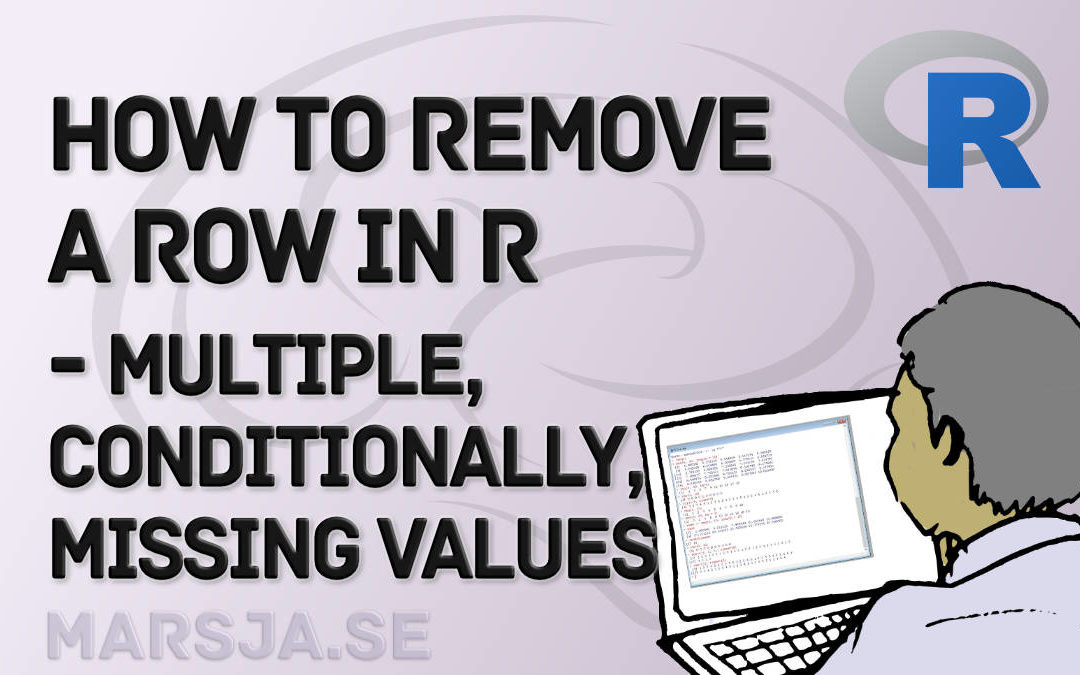## How to Remove/Delete a Row in R – Rows with NA, Conditions, Duplicated

In this tutorial, you will 1) learn how to delete a row in R, and 2) how to remove multiple rows in R. Of course, R being a versatile programming language there are many different options when we need to delete a row. For example, we can use the subset() function if...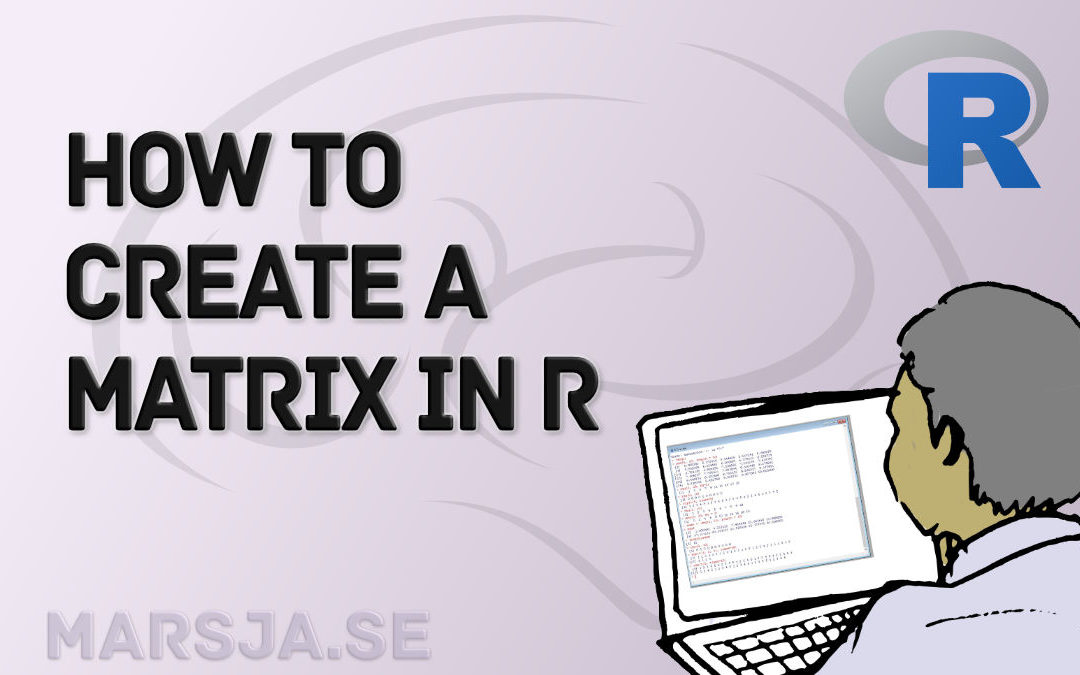## How to Create a Matrix in R with Examples – empty, zeros

In this short tutorial, you will learn how to create a matrix in R. We will use the matrix() function, among two other functions, for this aim. Specifically, we will go into the details of this function as this will enable us to e.g. name the columns and rows in the...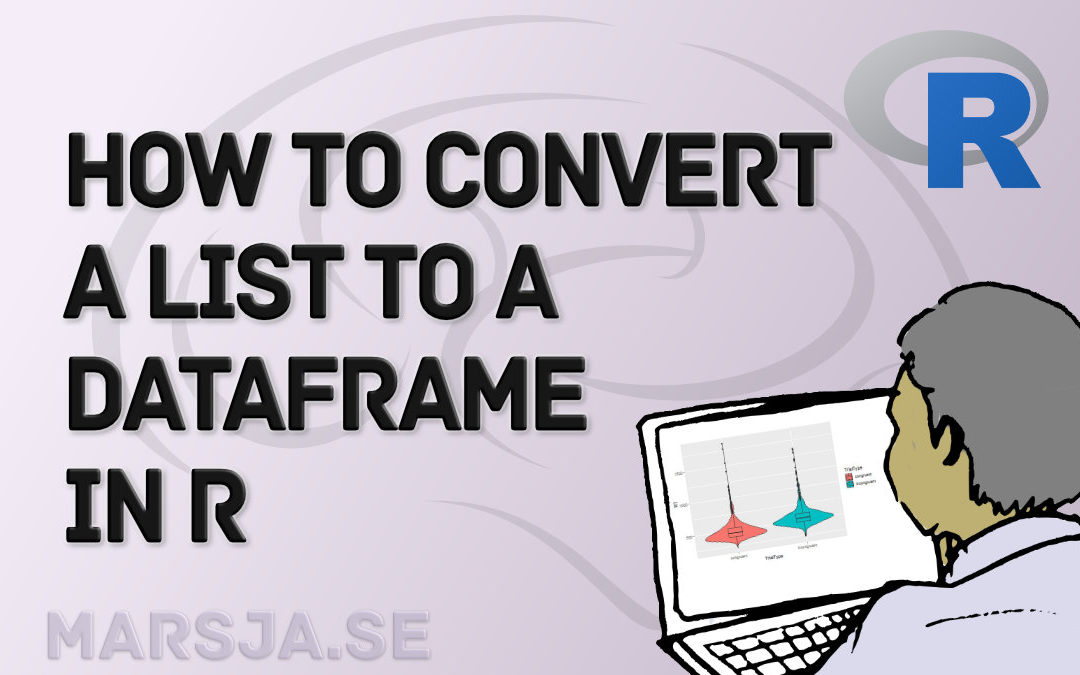## How to Convert a List to a Dataframe in R – dplyr

In this short tutorial, you will learn how to convert a list to a dataframe in R. To know how to convert lists to dataframes may be useful when you, for example, get your data from a source and they end up in a list of e.g. vectors. Here’s an example code template...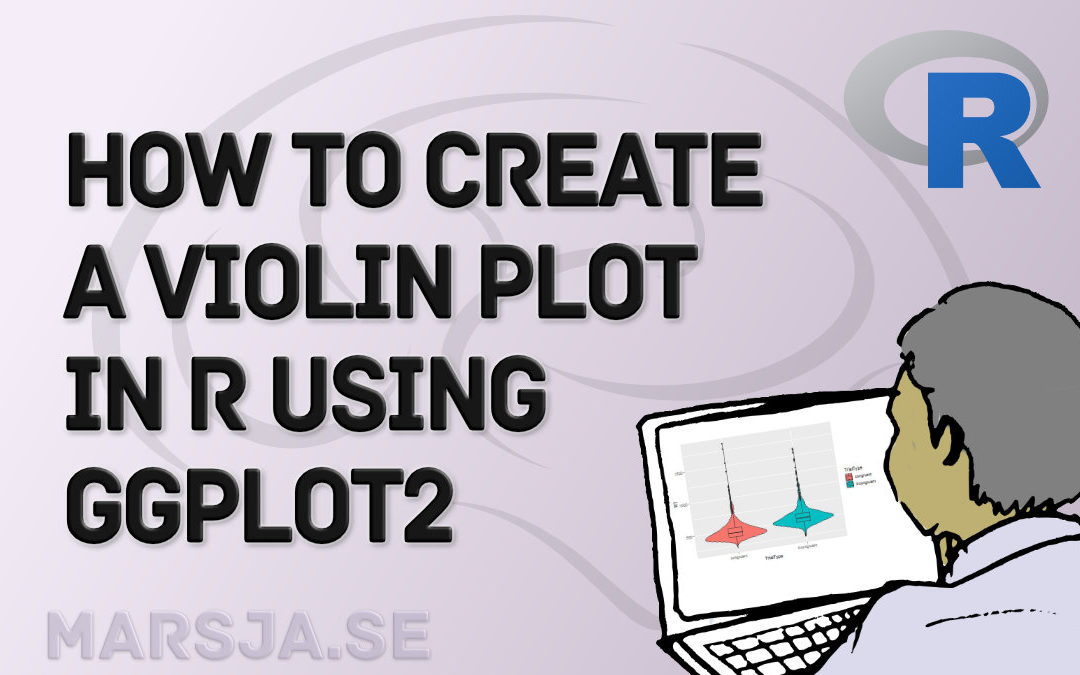## How to Create a Violin plot in R with ggplot2 and Customize it

In this data visualization tutorial, we are going to learn how to make a violin plot in R using ggplot2. Now, there are several techniques for visualizing data (see for example the Python-related post “9 Data Visualization Techniques You Should Learn in...## Learn How to Convert Matrix to dataframe in R with base functions & tibble

In this short tutorial, you will learn how to convert a matrix to a dataframe in R. Specifically, you will learn how to use base R and the package tibble to change the matrix to a dataframe. You will learn this task by 4 different examples (2 using each method). ...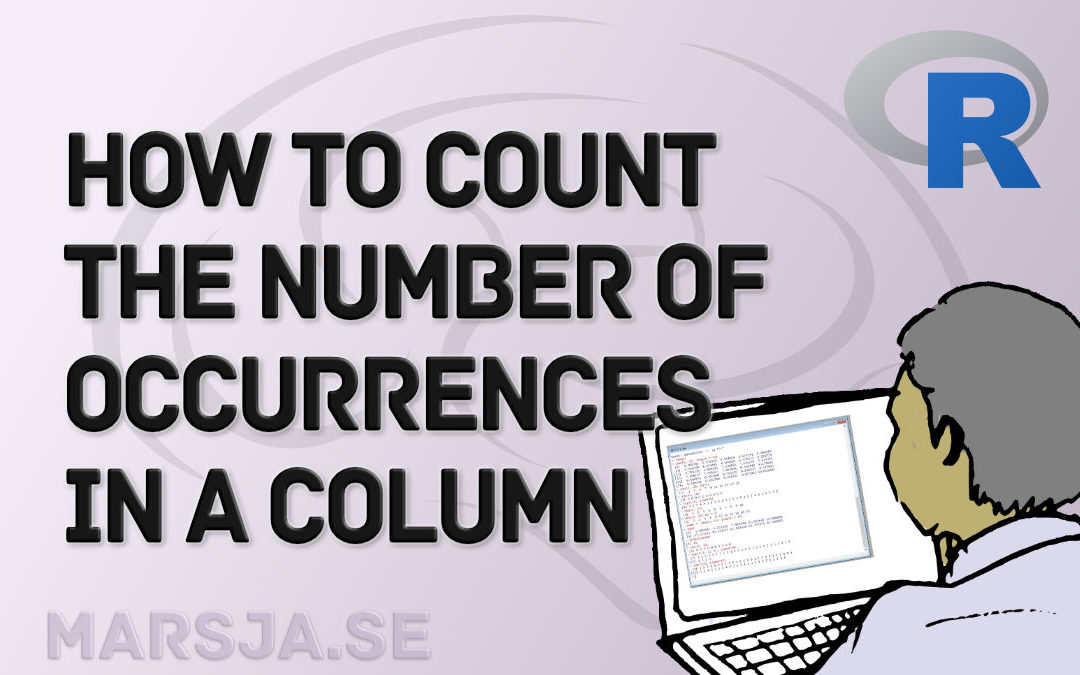## R Count the Number of Occurrences in a Column using dplyr

In this R tutorial, you are going to learn how to count the number of occurrences in a column. Sometimes, before starting to analyze your data,  it may be useful to know how many times a given value occurs in your variables. For example, when you have a limited...